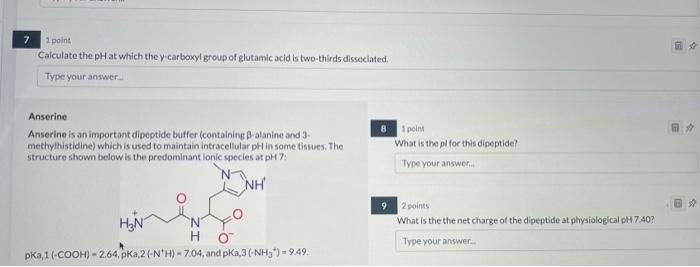# (Solved): $$7 \quad$$ 1point Calculate the pH at which the y carboxyl group of glutamic acid is two-thirds ...$$7 \quad$$ 1point Calculate the pH at which the y carboxyl group of glutamic acid is two-thirds dissociated. Type your answer_ Anserine Anserine is an important dipeptide buffer (containing $$\beta$$-alanine and 3 - 8 1 poins methylhistidine) which is used to maintain intracellular pH in some tissues. The What is the pif for this dipeptide? structure shown below is the predominant lonic species at pH 7 : 92 goints What is the the net charge of the dipeptide at phivsiological pH 7,40? pKa,1 $$(-\mathrm{COOH})=2.64, \mathrm{pKa}, 2\left(-\mathrm{N}^{+} \mathrm{H}\right)=7.04$$, and $$\mathrm{pKa}, 3\left(-\mathrm{NH}_{3}^{*}\right)=9.49$$

We have an Answer from Expert